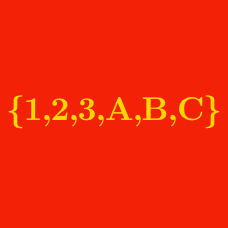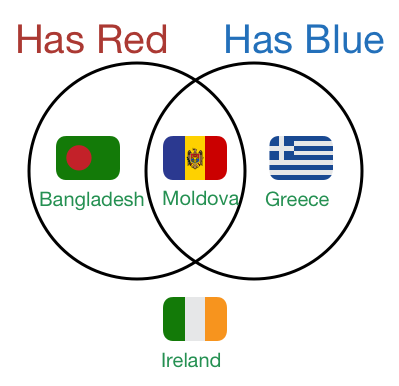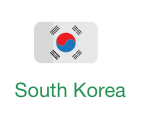Probability

# Sets - Problem SolvingRakesh is classifying flags of the world according to the presence of red or blue in them.

Which flag would be in the same category as that of South Korea?Given the sets \begin{aligned} U &=\{1,2,3,\ldots,8\},\\ A &=\{X \mid 8 \in X, X \subseteq U\},\\ B &=\{Y \mid 1 \in Y, Y \subseteq U\}, \end{aligned} what is $\lvert{A \cup B}\rvert?$

Details and assumptions

$\lvert{S}\rvert$ denotes the number of members of the set $S.$

Let $B$ be a subset of the set $A=\{1,2,3,4,5,\ldots,n\}$ such that $1$ and $2$ are elements in $B$ but $3$ and $4$ are not. If there are a total of $128$ such subsets $B$, what is the value of $n?$

In counting the numbers from 1 to 99 (inclusive), what is the total number of times that the digit 3 is used?

Details and assumptions
The number $12$ uses the digit 1 once and the digit 2 once.
The number $111$ uses the digit 1 three times.

Given the set $U=\{x \mid x \text{ is a positive integer } \leq 12\},$ let $A=\{2, 4\}$ and $B=\{2, 6, 9, 11, 12\}$ be two subsets of $U.$ How many sets $X$ are there such that \begin{aligned} \text{I}&. && X \subseteq U, \\ \text{II}&. && |B \cap X|=3, \\ \text{III}&. && A \text{ and } X \text{ are mutually disjoint?} \end{aligned}

Details and assumptions

You may choose to read the summary page Set Notation.

×